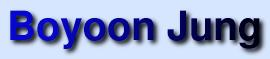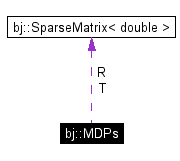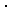Boyoon Jung's Library Manual

# bj::MDPs Class Reference [Markov Decision Process]

A Markov Decision Process Solver using a sparse matrix. More...

`#include <mdp.h>`

Collaboration diagram for bj::MDPs:[legend]
List of all members.

## Public Member Functions

MDPs (unsigned int nstates, unsigned int nactions, SparseMatrix< double > *T=0, SparseMatrix< double > *R=0)
A constructor.
~MDPs (void)
A destructor.
void initialize (SparseMatrix< double > *T, SparseMatrix< double > *R)
Initialize MDP models.
unsigned int * value_iteration (double discount=0.9, double epsilon=0.01, double *v0=0, int max_iter=-1)
Value iteration algorithm.
unsigned int * policy (void)
Return an optimal policy.
double * value_function (void)
Return a value function.null.
SparseMatrix< double > & getT (void)
Return a transition probability matrix.
SparseMatrix< double > & getR (void)
Return a reward matrix.
unsigned int numStates (void)
Return the number of states.
unsigned int numActions (void)
Return the number of actions.

## Static Public Member Functions

void convert_S_SA (unsigned int nstates, unsigned int nactions, SparseMatrix< double > &t, SparseMatrix< double > &s, SparseMatrix< double > &sa)
Convert a state-only reward matrix into a state-action matrix.
void convert_SAS_SA (unsigned int nstates, unsigned int nactions, SparseMatrix< double > &t, SparseMatrix< double > &sas, SparseMatrix< double > &sa)
Convert a state-aciton-state reward matrix into a state-action matrix.

## Protected Member Functions

virtual void load_transition_matrix (void)
Load a transition probability matrix.
virtual void load_reward_matrix (void)
Load a reward matrix.
void bellman_operation (unsigned int *p, double *v, SparseMatrix< double > &t, SparseMatrix< double > &r, double *vt_1, double discount)
Apply the bellman operation.
double span_semi_norm (double *vt, double *vt_1, unsigned int size)
Compute the span semi-norm.
void array_swap (double *&a0, double *&a1)
Swap two arrays.
void array_swap (unsigned int *&a0, unsigned int *&a1)
Swap two arrays.
double array_max (double *a, unsigned int size, unsigned int &index)
Find a maximum value in an array.
double sub_matrix_min (SparseMatrix< double > &a, unsigned int rows, unsigned int columns, unsigned int depth, unsigned int index)
Find a minimum sub-matrix.

## Protected Attributes

int num_states
The size of state space.
int num_actions
The number of actions.
SparseMatrix< double > T
A transition probability matrix.
SparseMatrix< double > R
A reward matrix.
unsigned int * P
An optimal policy.
double * V
A value function.
double * Vt_1
A value function in the previous step.
double * Q
An action-value function.

## Detailed Description

A Markov Decision Process Solver using a sparse matrix.

MDPs class provides a MDP (Markov Decision Process) solver. It provides only value iteration algorithm. Since a transition probability matrix and a reward matrix are stored in sparse-matrix form, the required memory size is not as big as MDP class.

## Constructor & Destructor Documentation

 bj::MDPs::MDPs ( unsigned int nstates, unsigned int nactions, SparseMatrix< double > * T = `0`, SparseMatrix< double > * R = `0` )

A constructor.

Parameters:
 nstates The number of states. nactions The number of actions. T A transition probability matrix. If 0, load_transition_matrix() should be overloaded by an inheriting class. R A reward matrix. If 0, load_reward_matrix() should be overloaded by an inheriting class.

 bj::MDPs::~MDPs ( void )
 A destructor.

## Member Function Documentation

 double bj::MDPs::array_max ( double * a, unsigned int size, unsigned int & index ) ` [inline, protected]`
 Find a maximum value in an array.

 void bj::MDPs::array_swap ( unsigned int *& a0, unsigned int *& a1 ) ` [inline, protected]`
 Swap two arrays.

 void bj::MDPs::array_swap ( double *& a0, double *& a1 ) ` [inline, protected]`
 Swap two arrays.

 void bj::MDPs::bellman_operation ( unsigned int * p, double * v, SparseMatrix< double > & t, SparseMatrix< double > & r, double * vt_1, double discount ) ` [inline, protected]`
 Apply the bellman operation.

 void bj::MDPs::convert_S_SA ( unsigned int nstates, unsigned int nactions, SparseMatrix< double > & t, SparseMatrix< double > & s, SparseMatrix< double > & sa ) ` [static]`

Convert a state-only reward matrix into a state-action matrix.

Parameters:
 nstates The number of states. nactions The number of actions. t A transition probability matrix. s A state-only reward matrix. sa A state-action reward matrix.

 void bj::MDPs::convert_SAS_SA ( unsigned int nstates, unsigned int nactions, SparseMatrix< double > & t, SparseMatrix< double > & sas, SparseMatrix< double > & sa ) ` [static]`

Convert a state-aciton-state reward matrix into a state-action matrix.

Parameters:
 nstates The number of states. nactions The number of actions. t A transition probability matrix. sas A state-action-state reward matrix. sa A state-action reward matrix.

 SparseMatrix& bj::MDPs::getR ( void ) ` [inline]`
 Return a reward matrix.

 SparseMatrix& bj::MDPs::getT ( void ) ` [inline]`
 Return a transition probability matrix.

 void bj::MDPs::initialize ( SparseMatrix< double > * T, SparseMatrix< double > * R )

Initialize MDP models.

Parameters:
 T A transition probability matrix. R A reward matrix.

 virtual void bj::MDPs::load_reward_matrix ( void ) ` [inline, protected, virtual]`
 Load a reward matrix. This function should be overloaded by an inheriting class unless a reward matrix R is provided in a constructor.

 virtual void bj::MDPs::load_transition_matrix ( void ) ` [inline, protected, virtual]`
 Load a transition probability matrix. This function should be overloaded by an inheriting class unless a transition probability matrix T is provided in a constructor.

 unsigned int bj::MDPs::numActions ( void ) ` [inline]`
 Return the number of actions.

 unsigned int bj::MDPs::numStates ( void ) ` [inline]`
 Return the number of states.

 unsigned int* bj::MDPs::policy ( void ) ` [inline]`
 Return an optimal policy.

 double bj::MDPs::span_semi_norm ( double * vt, double * vt_1, unsigned int size ) ` [inline, protected]`
 Compute the span semi-norm.

 double bj::MDPs::sub_matrix_min ( SparseMatrix< double > & a, unsigned int rows, unsigned int columns, unsigned int depth, unsigned int index ) ` [inline, protected]`
 Find a minimum sub-matrix.

 double* bj::MDPs::value_function ( void ) ` [inline]`
 Return a value function.null.

 unsigned int* bj::MDPs::value_iteration ( double discount = `0.9`, double epsilon = `0.01`, double * v0 = `0`, int max_iter = `-1` )

Value iteration algorithm.

Parameters:
 discount A discount factor. [0 ~ 1] epsilon Search of an optimal policy. ]0 ~ 1] v0 An initial value function. max_iter Maximum number of iterations. If negative, a proper number of iterations is computed.

## Member Data Documentation

 int bj::MDPs::num_actions` [protected]`
 The number of actions.

 int bj::MDPs::num_states` [protected]`
 The size of state space.

 unsigned int* bj::MDPs::P` [protected]`
 An optimal policy.

 double* bj::MDPs::Q` [protected]`
 An action-value function.

 SparseMatrix bj::MDPs::R` [protected]`
 A reward matrix.

 SparseMatrix bj::MDPs::T` [protected]`
 A transition probability matrix.

 double* bj::MDPs::V` [protected]`
 A value function.

 double* bj::MDPs::Vt_1` [protected]`
 A value function in the previous step.

The documentation for this class was generated from the following file:
ResearchProjectsPublications

ToolsBjLib ManualPlayer/StagePyPlayerMicroStrain IMUGPS/DGPSParticle Filter

PersonalWelcomeCurriculum VitaeResumeContact

Outside LinksUSCUSC CS DeptCRESRobotics LabRESL